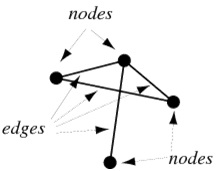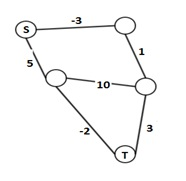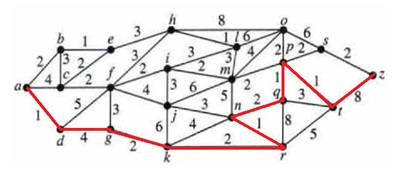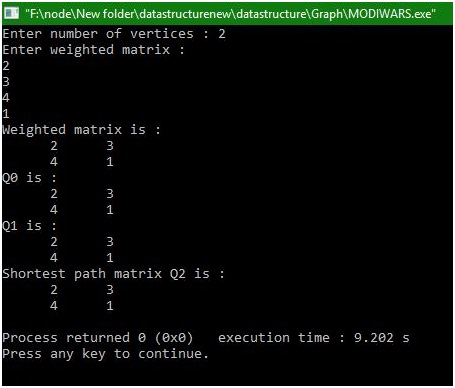# Modified Warshall's algorithm to find shortest path matrix

In this example, we will learn about weighted graph with positive and negative weights, shortest path and Warshall’s algorithm.
Submitted by Manu Jemini, on January 09, 2018Image source: http://mathworld.wolfram.com/images/eps-gif/GraphNodesEdges_1000.gif

A weighted graph with positive and negative weights can be understood as a graph with edges having cost. Edge is the line connecting two nodes or a pair of nodes. The cost can be positive or negative.Image source: https://i.stack.imgur.com/GlrNb.png

The shortest distance is the distance between two nodes. For Example, to reach a city from another, can have multiple paths with the different number of costs. A path with the minimum possible cost is the shortest distance.Image source: https://i.stack.imgur.com/tyTz7.png

Warshall’s algorithm is an algorithm which is used to find the shortest path between the source and destination nodes. These types of problems generally solved with BST if the cost of every edge is 1. But here the edges can have different values, even negative values. Hence we need to use this algorithm.

C program

```#include <stdio.h>
#define infinity 9999
#define MAX 20

int minimum(int a,int b)
{
if(a<=b)
return a;
else
return b;
}/*End of minimum()*/

int display(int matrix[MAX][MAX],int n )
{
int i,j;
for(i=0;i<n;i++)
{
for(j=0;j<n;j++)
printf("%7d",matrix[i][j]);
printf("\n");
}
}/*End of display()*/

main()
{
int i,j,k,n;

printf("Enter number of vertices : ");
scanf("%d",&n);

printf("Enter weighted matrix :\n");
for(i=0;i<n;i++)
for(j=0;j<n;j++)

printf("Weighted matrix is :\n");

/*Replace all zero entries of adjacency matrix with infinity*/
for(i=0;i<n;i++)
for(j=0;j<n;j++)
path[i][j]=infinity;
else

for(k=0;k<n;k++)
{
printf("Q%d is :\n",k);
display(path,n);
for(i=0;i<n;i++)
for(j=0;j<n;j++)
path[i][j]=minimum( path[i][j] , path[i][k]+path[k][j] );
}
printf("Shortest path matrix Q%d is :\n",k);
display(path,n);
}/*End of main() */
```

Output